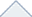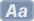###### Search the BibleKJVNKJVNLTNIVESVCSBNASB20NASB95NETRSVASVYLTDBYWEBHNV   RVR60VULWLCLXXmGNTTR [?]

Other Searches

###### Multi-Verse Retrieval
xKJVNKJVNLTNIVESVCSBNASB20NASB95NETRSVASVYLTDBYWEBHNV   RVR60VULWLCLXXmGNTTR
x
###### Daily Devotionals
x

Blue Letter Bible offers several daily devotional readings in order to help you refocus on Christ and the Gospel of His peace and righteousness.

x

Recognizing the value of consistent reflection upon the Word of God in order to refocus one's mind and heart upon Christ and His Gospel of peace, we provide several reading plans designed to cover the entire Bible in a year.

### Two-Year Plan

###### Recently Popular Media
x
Study Resources :: Dictionaries

# Dictionaries :: Ha(change letter)Search through a collection of ten unique Bible dictionaries and encyclopedias with quick results, using this Blue Letter Bible study tool. Find informative articles with definitions, word origins, links to verses and more, to boost your Bible study experience. Perfect for word searches and topical Bible studies.

Select the dictionary(ies) from which you would like to search.

a b c d e f g h i j k l m
n o p q r s t u v w x y z

We have searched through 10 Bible dictionaries and have yielded the following results for the letter you have chosen. For the purposes of speed, we have divided the results onto different pages. Please choose the first two letters of the word you are searching for from the list below.

Please choose the first two letters of the word you are searching for from the list below.

We have searched through 10 Bible dictionaries and have yielded the following results for the letter you have chosen.

Filter Results:

Ha [I,V]

Ha-Hiroth [I]

Ha-Jehudijah [I]

Haahashtari [H,I,N,B]

Habaiah [H,N]

Habakkuk [E,H,I,N,B]

Habazinaiah [H]

Habaziniah [I,N,B]

Habergeon [E,I,K,N,B]

Habitation [E,I,V]

Habor [E,H,I,N,B]

Hacaliah [I]

Hachaliah [H,N,B]

Hachilah [E,H,N]

Hachmoni [H,N,B]

Haemorrhoids [E]

Haft [E,K]

Hagab [H,I,N,B]

Hagaba [I,N,B]

Hagabah [I,B]

Hagar [E,H,I,N,B]

Hagarene [E]

Hagerite [I]

Haggai [E,H,I,N,B]

Haggeri [H,I,N,B]

Haggi [I,N,B]

Haggiah [H,I,N,B]

Haggites [I]

Haggith [E,H,I,N,B]

Hagia [I]

Hagiographa [E,I]

Hagri [I]

Hagrites [I]

Hai [I,N,B]

Hail [E,K,N]

Hail (1) [I]

Hail (2) [I]

Hail (Noun) [V]

Hail (Verb) [V]

Hail! [E]

Hair [E,I,N,V,B]

Hair, The [T]

Hakkatan [H,I,N,B]

Hakkoz [E,H,I,N,B]

Hakupha [H,I,N,B]

Halah [E,H,I,N,B]

Halak [E,H,N,B]

Halak, Mount [I]

Halakha [I]

Hale [K]

Hale (Verb) [V]

Hale; Haling [I]

Half [I,V]

Half-Homer [N]

Half-Shekel [V]

Halhul [E,H,I,N,B]

Hali [H,I,N,B]

Hall [E,I,V,B]

Hallel [E,I]

Hallelujah [E,H,I,N,V,B]

Halloesh [H]

Hallohesh [I,B]

Hallow [E,K,V]

Halohesh [N,B]

Halt [E,I,K,V]

Haltil [B]

Ham [E,H,N,B]

Ham (1) [I]

Ham (2) [I]

Haman [E,H,I,N,B]

Hamath [E,H,I,N,B]

Hamath-Zobah [E,H,I,N]

Hamathzobah [B]

Hammath [E,I,N,B]

Hammedatha [E,H,I,N,B]

Hammelech [E,H,I,N,B]

Hammer [E,I,N]

Hammolecheth [I]

Hammoleketh [E,N,B]

Hammon [E,H,I,N,B]

Hammoth-Dor [E,I,N]

Hammothdor [B]

Hammuel [I]

Hammurabi [I]

Hamon [E]

Hamon-Gog [E,H,I,N]

Hamonah [E,H,I,N,B]

Hamongog [B]

Hamor [E,H,I,N,B]

Hamoth [H]

Hamran [I]

Hamuel [I,N,B]

Hamul [E,H,I,N,B]

Hamutal [E,H,I,N,B]

Hanameel [E,H,B]

Hanamel [I,N]

Hanan [E,H,I,N,B]

Hananeel [E,H,N,B]

Hanani [E,H,I,N,B]

Hananiah [E,H,I,N,B]

Hananiel [B]

Hand [E,I,N,V]

Hand Weapon [I]

Handed Down [V]

Handful [I]

Handicraft [I,B]

Handkerchief [E,I,N,V]

Handle [I,V]

Handmaid [E,I]

Hands, The [T]

Handstaff [I]

Handwriting [E,I,V]

Hanes [E,H,I,N,B]

Hang [V]

Hanging [E,I,N]

Hangings [I,N]

Haniel [H,I,B]

Hannah [E,H,I,N,B]

Hannathon [H,I,N,B]

Hanniel [E,H,I,N]

Hanoch [H,N,B]

Hanun [E,H,I,N,B]

Hap; Haply [I]

Hapharaim [H,I]

Haphraim [N,B]

Haply [K]

Happen [I,V]

Happiness [I,N]

Happizzez [I]

Har-Magedon [I]

Hara [E,H,I,N,B]

Haran [E,H,N,B]

Haran (1) [I]

Haran (2) [I]

Hararite [I,B]

Harbona [E,N,B]

Harbonah [H,B]

Harbour [I]

Hard By [K]

Harden [I]

Hardly [V]

Hare [E,I,N,B]

Harem [N,B]

Hareph [H,I,N,B]

Hareth [E,I,N,B]

Harhaiah [E,H,I,N,B]

Harhas [H,I,N]

Harhur [E,H,I,N,B]

Harim [E,H,I,N,B]

Hariph [E,I,N,B]

Harlot [E,I,V,B]

Harlotry [I,N]

Harm [V]

Harmagedon [B]

Harmless [V]

Harnepher [E,H,I,B]

Harness [E,I]

Harod [E,H,N,B]

Harodite [E,I]

Haroeh [I,N,B]

Harorite [I,B]

Harosheth [H,N,B]

Harp [E,I,N,V,B]

Harper [V]

Harphite [B]

Harran [H]

Harrow [E,I,K,N,B]

Harrows [I]

Harsha [E,H,I,N,B]

Harsith [I]

Hart [E,I,K,N,B]

Hart, The [T]

Harum [E,H,I,N,B]

Harumaph [H,I,N,B]

Haruphite [E,H,I]

Haruz [E,H,I,N,B]

Harvest [E,I,N,V,B]

Harvest, The [T]

Hasenuah [E,I,N,B]

Hashabiah [E,H,I,N,B]

Hashabnah [H,I,N,B]

Hashabneiah [I]

Hashabniah [E,N,B]

Hashem [H,I,N,B]

Hashmonah [E,I,N,B]

Hashub [E,H,I,N,B]

Hashubah [E,H,I,N,B]

Hashum [E,H,I,N,B]

Hashupha [H,N,B]

Hasidaeans [I]

Hasmoneans [I]

Hasrah [E,H,I,N,B]

Hassenaah [I,B]

Hassenuah [I]

Hasshub [I,B]

Hassophereth [I]

Haste [I,K,N]

Hasupha [E,I,N,B]

Hat [E,I,N]

Hatach [E,H,I,N,B]

Hatchet [I]

Hate; Hatred [I]

Hathach [I]

Hathath [E,H,I,N,B]

Hatipha [E,I,N,B]

Hatita [E,H,I,N,B]

Hatred [E,N,T]

Hattil [H,I,N]

Hattipha [H]

Hattuph [B]

Hattush [E,H,I,N]

Haughtiness [N]

Haughty [K,V]

Haunt [I,K]

Hauran [E,H,I,N,B]

Have [I,V]

Haven [E,I,V]

Havens, Fair [I]

Havilah [E,H,I,N,B]

Havoc [I,V]

Havoth-Jair [E,H,N]

Havothjair [B]

Havvah [I]

Havvoth-Jair [I]

Hawk [E,I,N,B]

Hay [E,I,N,V,B]

Hazael [E,H,I,N,B]

Hazaiah [H,I,N,B]

Hazar [I]

Hazar-Enan [E,N]

Hazar-Hatticon [E,H,N]

Hazar-Maveth [E]

Hazar-Shual [E,H,N]

Hazar-Susah [E,H,N]

Hazard [V]

Hazarenan [H]

Hazarmaveth [H,N,B]

Hazel [E,I,N,B]

Hazelelponi [H,I,N,B]

Hazer [B]

Hazerim [E,I,N,B]

Hazeroth [E,H,I,N,B]

Hazezon-Tamar [E,H,I,N]

Hazezontamar [B]

Haziel [I,N,B]

Hazo [E,H,I,N,B]

Hazor [E,H,I,N,B]